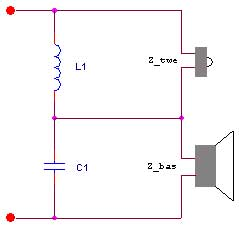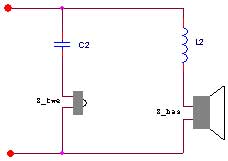# Serial or Parallel Filtering

Series filtering or parallel filtering, -which is the best choice in loudspeakers networks?
There is no single sided answer to this question; rather, the application is what decides the answer.

### Series filtering

In the following, the electrical network illustrated beneath is considered.In series filtering scenarios, the loudspeaker units are connected in series, which means that electrical noise caused in the feedback voltage influences the other loudspeakers in the network.
This effectively means that the performance of series filtering scenarios directly depends on the electrical characteristic of the loudspeaker units and that an electrical unity gain is ALWAYS ensured (provided that the filter components are lossless). Conversely, the voice coil of each loudspeaker is included as filter components, which effectively means that odd rolloff slopes of approximately 9 dB/octave are obtained, due to the semi-inductive nature of the voice coil.

### Parallel filtering

The parallel filtering scenario offers the possibility of sophisticated solutions where a filter for each loudspeaker can be hand sewn. Many different pre-defined filter characteristics can be designed using relatively simple analytical solutions, since only ONE loudspeaker is included for each of these filters.
In the case of a first order parallel filter scenario (as illustrated below) a Butterworth characteristic is obtained.### Quality Considerations!

Parallel filters are described by the Q value, which reveal the amount of voltage attenuation at the crossover frequency. For Q = 1 the attenuation is 0 dBV at the crossover frequency, for Q = 0.707, there is an attenuation of 3 dBV at the crossover frequency and for Q = 0.5 the voltage is attenuated 6 dBV at the crossover frequency.
The serial filters are described by the Zeta value. Zeta = 1.0 yields the standard Butterworth (-3dBV at the crossover frequency), Zeta = 0.5 is under damped and yield the "quasi-second order" crossover and Zeta = 2 represents an overdamped filter.